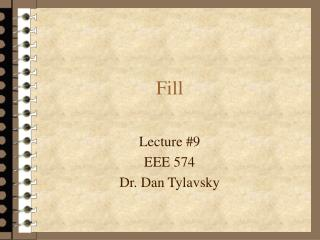DownloadDownload PresentationFill

# Fill

Télécharger la présentation## Fill

- - - - - - - - - - - - - - - - - - - - - - - - - - - E N D - - - - - - - - - - - - - - - - - - - - - - - - - - -
##### Presentation Transcript

1. Fill Lecture #9 EEE 574 Dr. Dan Tylavsky

2. When we solve Ax=b, (A sparse), fill-in will occur in the L,U factor. • Defn: Fill (or Fill-in) - is the creation of non-zero’s in positions in the L/U factor that are zero in the A matrix. • EX: Table of Factors* *L,D,U Indicate positions of nonzero in original matrix. z Indicates positions where fill occurs.

3. By changing the ordering we can reduced the fill. • Re-label nodes so that orig. node 1 <=> orig. node 7. Table of Factors • We will look at ways of ordering matrices so that we can minimize fill. For now we need to be aware that fill will occur and be able to account for it when creating the data structure for the LU factors.

4. Fill-in Lemma: The non-zeros in row k = {native non-zeros in row k} } { non-zeros (including fill) in row numbers corresponding to col. indices of non-zero’s to left of diagonal (including fill) of row k. Xp=Native non-zero in row p. Fp = Fill cause by non-zero from inactive row p.

5. * *D,T,X,F are native non-zeros or fill produced by the triangular factorization process. Fill-in Theorem: When determining fill of row k, of a incidence- symmetric matrix, it is sufficient, to take into account only those rows (of index less than k) for which the non-zero in column k is the first non-zero of the row to the right of the diagonal. I.e., For the matrix shown below (assuming all values not shown in rows i, j, k, are zero) only the non-zero’s (including fill) in row j need be used when estimating the fill in row k -- even though row i is used in the reduction of row k.

6. Proof: Prove that row i with lowest-number right-of-diagonal element <k, need not be considered when contributing fill to row k. 2. Because of symmetry Xi Xk, (Xi not lowest-numbered right-of-diag.entry in row i.) 3. Because of Xk, row i will contribute fill to row k. 4. Because of symmetry Ti Tj , (Ti is lowest-numbered right-of-diag. entry in row i.) 5. Because of Tj, row i will contribute fill to row j. 6. Because row i contributes fill to both row j and row k, Fji, Fki will be created , (Fji is lowest-numbered above-diag. entry in row j.) 7. Because Fki exists, row j will contribute fill to row k. 8. From Lemma 1, row j has non-zeros everywhere row i does (plus it’s own native nonzeros). 9. Hence, non-zeros of row i need not be accounted for when determining fill of row k. D,T,X are native entries in matrix. Fmi is fill in row m due to row i. Ti is lowest number above diagonal entry in row i.

7. Fill Corollary I: The sets of rows needed to account for fill to an arbitrary row, Sk, are mutually disjoint, i.e., • Rows needed to account for fill to row k have their lowest numbered right-of-diagonal entry in column k. • There is only one lowest-numbered right-of- diagonal entry for each row. • Therefore: rows in Si will not occur in any other set. Fill Corollary II: The members of each set of rows needed to account for fill to an arbitrary row Sk, are not duplicated. • Each row has one and only one lowest numbered right-of- diagonal element. • Therefore: no duplicate entries in Si.

8. (Fill Corollary II) Because there are no duplicate entries in the each set of nodes, Si, we can use a self referential linked list for the set of nodes that contribute fill to each node. • (Fill Corollary I) Because the sets, Si, are mutually disjoint, we need only one self referential linked list to store all Si.

9. 1 4 3 7 5 6 2 Ex: Assuming no pivoting, determine the fill that will occur during LU factorization of the matrix shown below, and construct the sets, Si, that contain the minimum list of row numbers needed to account for fill in row i. R1 contributes fill to row 4. R2 contributes fill to row 6. R3 contributes fill to row 4. R4 contributes fill to row 5. R5 contributes fill to row 6.

10. Team Problem: Determine the fill that will occur during LU factorization of the matrix shown below, and construct the sets, Si, that contain the minimum list of row numbers needed to account for fill in row i.

11. Team Problem: Sketch a schematic diagram of the topology of the matrix from the previous slide.

12. The End